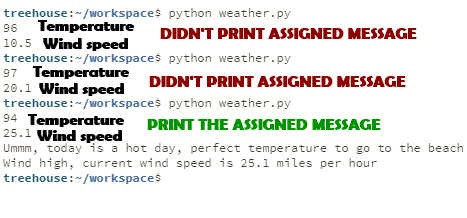## Welcome to the Treehouse Community

Want to collaborate on code errors? Have bugs you need feedback on? Looking for an extra set of eyes on your latest project? Get support with fellow developers, designers, and programmers of all backgrounds and skill levels here with the Treehouse Community!

### Looking to learn something new?

Treehouse offers a seven day free trial for new students. Get access to thousands of hours of content and join thousands of Treehouse students and alumni in the community today.```import random
degrees_f = random.randint(60, 110)
wind_speed = round(random.uniform(5.0, 30.0), 1)

print(degrees_f)
print(wind_speed)
#If and else for temp in F degrees
if degrees_f >= 60 and degrees_f <= 70:
temp_broadcast = "It might be a little cold today, you might want to put on some coat"
if degrees_f >= 71 and degrees_f <= 86:
temp_broadcast = "It looks like today might be a good day for a walk in the park"
if degrees_f >= 87 and degrees_f <= 110:
temp_broadcast = "Ummm, today is a hot day, perfect temperature to go to the beach"
#If and else for wind speed
if wind_speed >= 5.0 and wind_speed <= 10.0:
wind_broadcast = "Wind low, current wind speed is {} miles per hour".format(wind_speed)
if wind_speed >= 11.0 and wind_speed <= 20.0:
wind_broadcast = "Wind medium, current wind speed is {} miles per hour".format(wind_speed)
if wind_speed >= 21.0 and wind_speed <= 30.0:
wind_broadcast = "Wind high, current wind speed is {} miles per hour".format(wind_speed)

```

So what I am trying to do is get a random int for "degrees_f" and a round float for "wind_speed". Then I have assigned some if statements for each number, for example, this:

```if degrees_f >= 60 and degrees_f <= 70:
temp_broadcast = "It might be a little cold today, you might want to put on some coat"
```

or this:

```if wind_speed >= 21.0 and wind_speed <= 30.0:
wind_broadcast = "Wind high, current wind speed is {} miles per hour".format(wind_speed)
```

But the problem that I am having is that sometimes It would generate random numbers but it would not put out the assigned messages. If you look at the top you would see that I have this code there so I can see what random numbers have been generated:

```print(degrees_f)
print(wind_speed)
```

So here is an example how this works, if "degrees_f" generated the number 72, so if you look at my if statements that means that it should put out this message, "It looks like today might be a good day for a walk in the park", but sometimes it does not and I'm not sure why.

Sorry for the confusing, I'm pretty new to Python and I don't even know how to explain the problem that I'm having. If you did not understand what is my problem, please code the first code that I have posted and try it out and you will see that sometimes it would not prompt the assigned message.

Here is a picture of how sometimes it does not put out the assigned message:Hopefully somebody can help me with this problem, thanks !

your windspeed is not an integer so it will fall out at 10.5 and 20.1.

```if wind_speed >= 5.0 and wind_speed <= 10.0:
if wind_speed >= 11.0 and wind_speed <= 20.0:
if wind_speed >= 21.0 and wind_speed <= 30.0:
```

any values between 10 and 11 as well as any values between 20 and 21 will not meet the criteria. for any of your wind speed.

1. either convert wind_speed to integer or
2. try new logic
```    if wind_speed >= 5.0 and wind_speed <= 10.0:
if wind_speed > 10.0 and wind_speed <= 20.0:
if wind_speed > 20.0 and wind_speed <= 30.0:
```